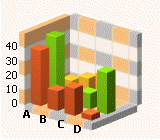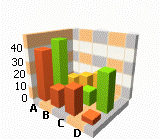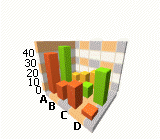21.2
21.1
20.2
20.1
19.2
19.1
18.2
18.1
17.2

Diagram3D.PerspectiveAngle Property

Gets or sets the perspective angle for a 3D diagram in a perspective projection (when Diagram3D.PerspectiveEnabled is true).

Namespace: DevExpress.XtraCharts

Assembly: DevExpress.XtraCharts.v21.2.dll

Declaration

[XtraChartsLocalizableCategory(XtraChartsCategory.Behavior)]
public int PerspectiveAngle { get; set; }

Property Value

Type Description
Int32

An integer value that may be 0 and more, but less than 180.

Remarks

In a perspective projection the lines that are parallel to the object converge as they move towards a theoretical horizon line on the projection surface. The rate at which parallel lines converge is called the perspective angle. This angle is determined by the distance of an imaginary viewer from a 3D diagram object.

See the table below which illustrates the 3D chart with different perspective angles.

PerspectiveAngle = 0 PerspectiveAngle = 50 PerspectiveAngle = 90Note

If the PerspectiveAngle property value is set to 0, the perspective projection is disabled (the same as if Diagram3D.PerspectiveEnabled property is set to false.)